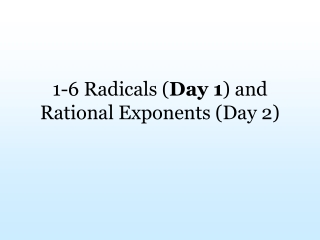DownloadDownload Presentation1-6 Radicals ( Day 1 ) and Rational Exponents (Day 2)

# 1-6 Radicals ( Day 1 ) and Rational Exponents (Day 2)

Download Presentation## 1-6 Radicals ( Day 1 ) and Rational Exponents (Day 2)

- - - - - - - - - - - - - - - - - - - - - - - - - - - E N D - - - - - - - - - - - - - - - - - - - - - - - - - - -
##### Presentation Transcript

1. 1-6 Radicals (Day 1) and Rational Exponents (Day 2)

2. What is a Radical? In simplest term, the definition of the radical ( ) is this: If a > 0 then where x > 0 and = Principle Square Root = The Positive root

3. Now extend to when : The Principle nth root of a: • If a > 0 then =x such that 2. 3. If a < 0 and n is odd then is a negative number such that . 4. If a < 0 and n is even, is not real.

4. AUGH! What was all that? Vocab Word That was the Mathlish for evaluating radical expressions where the index ≠ 2. Lets learn the vocabulary before we practice the problems. Radical Expression Index or Root Radicand ***If n is missing, then it is understood to be 2

5. Form of a Radical Problem because because Notice this method!!

6. Rules of Radicals So, the goal today will be to pull out perfect roots (perfect squares, cubes, etc). Prime factoring will often help.

7. Practice problems Simplify each expression